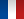• #### Number of hours

• Lectures 15.0
• Projects -
• Tutorials 15.0
• Internship -
• Laboratory works -
• Written tests 3.0

ECTS 2.5

## Goal(s)

• This is an introductory course on the basic techniques of mathematical programming. It is a basic course in linear programming in continuous variables.
• At the end of the course, students will be able to model a simple problem using a linear program, including translating the constraints of the problem through a system of linear equations or inequalities. Students will also be able to solve the program using the simplex algorithm and give different economic interpretations of the output of this algorithm, using the duality theory.
For more complex problems, students will know how to model it by a linear integer or mixed program. Resolution methods such as Branch & Bound algorithm and the quality of the models will be studied to solve these problems efficiently.
• Students will use linear solvers such as Excel and OPL Studio.

Responsible(s)

Olivier BRIANT

## Content(s)

The main points are

• Modelling a problem in a linear program
• Resolution algorithms: geometrics, simplex and variants
• Duality and economic interpretation
• Sensitivity analysis
• Modelling a problem in linear programming in mixed or integer variables
• Branch & Bound algorithm
• Quality of the various modellisations
• Modelling logical constraints
• Using commercial solver

Prerequisites

Basic courses on linear algebra and matrices.

Test

Two written exams 1:30, accounting respectively for 60% and 40% of the rating of the EU.
Authorized documents: GI calculator and handwritten double-sided A4 paper.

N1 = 0.4*CC + 0.6*E1

N2 = E2

Calendar

The course exists in the following branches:

• Curriculum - Engineer IPID apprentice program - Semester 6
see the course schedule for 2023-2024

Course language(s):